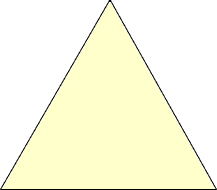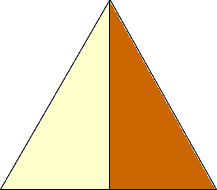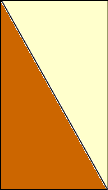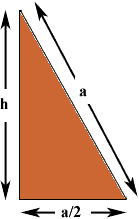Quandaries and Queries Can you help me understand why the area of a equilateral triangle is the square root of 3 divided by 4 times the lenght of the side squared? student Hi, I drew a diagram of an equilateral triangle.Draw a line from the vertex to the midpoint of the base.Cut along this line and move half of the triangle to form a rectangle.If a is the length of a side of the equilateral triangle and h the height then this rectangle has area  1/2ah The triangle below, which is half of the rectangle, is a right triangle,and hence, by Pythagoras' theorem a2 = h2 + ( a/2)2 Thus h = sqrt( 3/4 a2) =  sqrt(3)/2 a. Hence the area of the triangle is  1/2ah = sqrt(3)/4 a2 Penny Go to Math Central